0
283

# LIC AAO Expected Questions PDF

Question 1: Select the one which is best express the same sentence in Passive Voice.
They drew a circle in the morning.

a) A circle was being drawn by them in the morning

b) A circle was drawn by them in the morning

c) In the morning a circle have been drawn by them

d) A circle has been drawing since morning

Question 2: Out of the four alternatives suggested, select the one which best express the same sentence in passive/Active Voice.
We must respect the elders.

a) The elders deserve respect

b) The elders must be respected

c) The elders must be respected by us

d) Respect the elders we must

Question 3: Influenza: Virus: Typhoid:?

a) Bacillus

b) Parasite

c) Protozoa

d) Bacteria

Question 4: Analogy for
ISLAND : ARCHIPELAGO ::

a) shoulder : arm

b) stomach : intestine

c) shoe : laces

d) toe : foot

Question 5: A papaya tree was planted 2 years ago. It increases at the rate of 20% every year. If at present, the height of the tree is 540 cm, what was it when the tree was planted ?

a) 324 cm

b) 400 cm

c) 375 cm

d) 432 cm

Question 6: A cube is painted on all sides using white and red colours such that opposite faces are painted in different colours. This cube is cut into 27 smaller cubes of equal size.
How many smaller cubes will have only one face coloured ?

a) 3

b) 6

c) 8

d) 12

Question 7: The perimeter of a circular and a square field are equal. What is the diameter of the circular field, if the area of the square field is 484 $metre^{2}$

a) 14 metre

b) 21 metre

c) 28 metre

d) None of these

Question 8: The difference between two numbers is 14 and one-fourth of the sum of the number is equal to 22. Find the numbers.

a) 23 and 37

b) 33 and 55

c) 37 and 51

d) 40 and 54

e) None of these

Question 9: A, B, C started a shop by investing Rs. 27000, Rs. 81000 and Rs. 72000 respectively. At the end of one year, B’s share in total profit was Rs. 36000. What was the total profit ?

a) Rs. 108000

b) Rs. 116000

c) Rs. 80000

d) Rs. 92000

Question 10: A dealer offers a discount of 10% on the marked price of an article and still makes a profit of 20%. If its marked price is Rs. 800, then the cost price is:

a) Rs. 600

b) Rs. 700

c) Rs. 800

d) Rs. 900

Instructions

A town has 832 residents. Three newspapers operate in this town – Times of India, The Hindu, and Indian Express. 496 residents ordered The Hindu. Exactly 108 residents did not order any newspaper. 16 residents ordered all three newspapers and 192 residents ordered Indian Express. The number of residents who ordered only Indian Express and The Hindu is equal to the number of residents who ordered Times of India and The Hindu. The number of residents who ordered only Indian Express and The Hindu is twice the number of residents who ordered only Indian Express and Times of India, which in turn is twice the number of residents who ordered all three newspapers.

Question 11: How many residents ordered more than one newspaper?

a) 192

b) 176

c) 160

d) 144

Question 12: If ‘-’ stands for division, ‘+’ stands for subtraction, ‘$\div$’ stands for multiplication, ‘$\times$’ stands for addition, then which one of the following equations is correct ?

a) $70-2+4\div5\times6=44$

b) $70-2+4\div5\times6=21$

c) $70-2+4\div5\times6=341$

d) $70-2+4\div5\times6=36$

Question 13: (i) Six friends A, B, C, D, E and F are seated in a circle facing each other.
(ii) A is between D and B and F is between C and E
(iii) C is the third to the left of B
Which of the following is the position of A in relation to F ?

a) Second to the left

b) Second to the right

c) third to the right

d) Fourth to the left

Question 14: There are five different houses, A to E, in a row. A is to the right of B and E is to the left of C and right of A. B is to the right of D.A is to the left of E. Which of the houses is in the middle ?

a) A

b) E

c) D

d) C

Instructions

Below are two statements followed by two conclusions. Assume these statements are true irrespective of whether they are true in real life.

Question 15: Statements: All cells are phones. Some phones are wireless. All wireless are devices.
Conclusion I: Some cells are devices
Conclusion II: Some devices are phones

a) If only conclusion I follows

b) If only conclusion II follows

c) If either conclusion I or II follows

d) If neither conclusion I or II follows

e) If both conclusion I and II follow

Question 16: Statements: All musicians are music lovers. No music lover likes drums.
Conclusion I: No musician likes drum
Conclusion II: All music lovers are musicians

a) If only conclusion I follows

b) If only conclusion II follows

c) If either conclusion I or II follows

d) If neither conclusion I or II follows

e) If both conclusion I and II follow

Instructions

The given Venn diagram shows the categories of people who are Rock Stars, Pop Stars and Hip Stars. Study the diagram carefully and answer the questions that follow: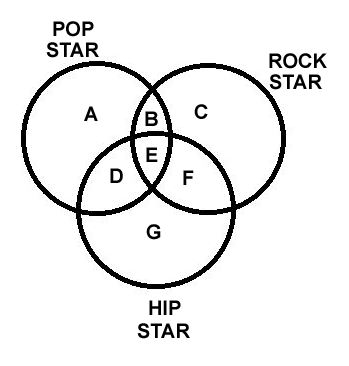Question 17: What is the total number of Rock Stars in the diagram?

a) Only C

b) C + B

c) C+ B + F

d) C+ B + E + F

e) C+B+E+F+ D

Question 18: Which area represents Rock Stars who are Pop Stars but not Hip Stars?

a) A

b) C

c) G

d) B

e) F

Question 19: In a dice a, b,c and d are written on the adjacent faces in a clockwise order and e and f are at the top and bottom of cube. When c is at the top, what will be at the bottom ?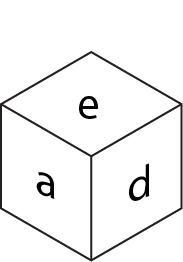a) c

b) a

c) e

d) b

Question 20: Find the missing figure from the given alternatives :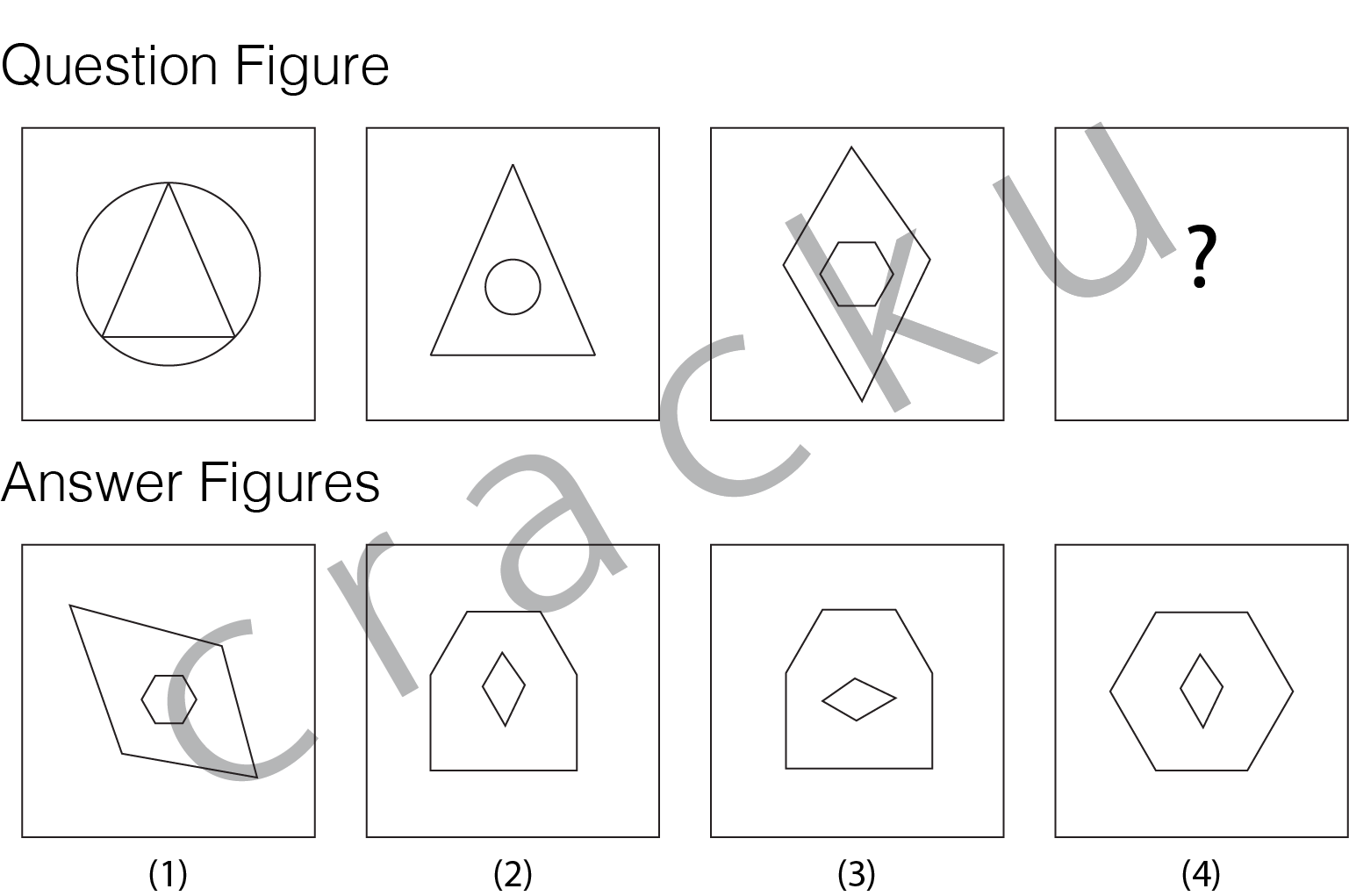a) 1

b) 2

c) 3

d) 4

Here, in active voice ‘they’ is subject and ‘circle’ is object.
Hence, circle will get priority in passive voice.
Therefore, passive voice is:
A circle was drawn by them in the morning.

The correct option is option B.

Here, ‘We’ is subject while ‘elders’ is the object.
Therefore, to convert to passive voice the object will have to take active role.
Hence, the sentence in passive voice would read as:
The elders must be respected.
Therefore, the correct option is option B.

Here, the coorelation is between the disease and it’s vector.
Influenza’s vector is virus. Typhoid’s vector is bacteria.
Therefore, the correct option is option D.

Archipelago means a group of islands. Island thus forms a part of archipelago.
Similarly, toe is part of foot.
Therefore, the correct pair having similar relationship is foot-toe.
Hence, the correct option is option D.

This problem can be viewed as that of compound interest.
Here, the trees growth is componded each year.
Now, let the original height be x.
540 = x*(1.2)^2
x = 375
Hence, the trees height was 375cm before 2 years.

On each face there is only one cub whose only one side is exposed outside.
The cube has 6 faces and hence there will be 6*1 = 6 cubes whose only one side will be painted.
Therefore, the correct option is option B.

Let the square of side be x,
Now area = 484
x^2 = 484, x=22
Perimeter = 4*22= 88
Circumference = 88
2*(22/7)*r = 88
Hence, r=14
Area= (22/7)*14*14 =616
Therefore, option C is correct.

A, B and C will recieve profits in proportion to their investments.
Now, their investments are in the ratio 3:9:8
Let the common multiple be x.
Now, B’s share = 36000
Therefore, (9/20)x = 36000
x = 80,000
The correct option is option C.

Dealer has offered a discount of 10% on his marked price of 800.
Therefore, selling price = 800 – 80 = 720
Percent Profit = $\frac{Selling Price – Cost Price}{Cost Price}$*100
0.2 = $\frac{720 – Cost Price}{Cost Price}$
Cost Price = 720/1.2
Cost Price = 600
Therefore option A is correct.

It is given that the number of residents who ordered only Indian Express and Times of India is twice the number of residents who ordered all three newspapers.

So, the number of residents who ordered only Indian Express and Times of India is equal to 2*16 = 32.

It is also given that the number of residents who ordered only Indian Express and The Hindu is twice the number of residents who ordered only Indian Express and Times of India.

So, the number of residents who ordered only Indian express and The Hindu is equal to 2*32 = 64.

It is given that the number of residents who ordered only Indian Express and The Hindu is equal to the number of residents who ordered Times of India and The Hindu.

The number of residents who ordered only Indian Express and Hindu is equal to 64. So, the number of residents who ordered Times of India, The Hindu and at most one more newspaper is equal to 64. So, the number of residents who ordered only Times of India and The Hindu is equal to 64 – 16 = 48.

So, the number of residents who ordered more than one newspaper = 32 + 64 + 48 + 16 = 160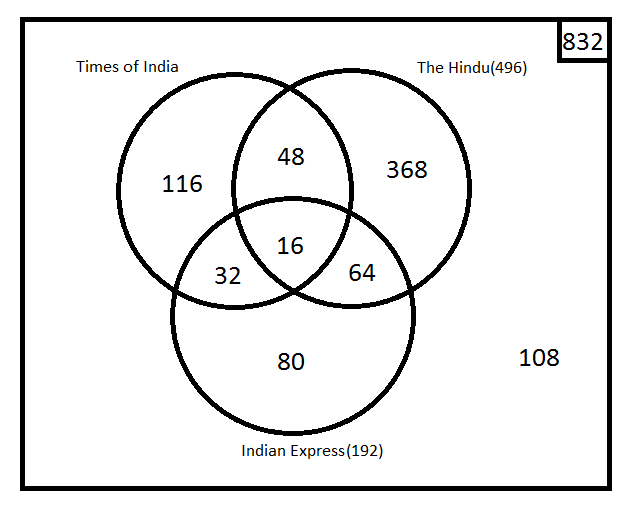We have to analyse each and every expression with the correct symbols,
$70-2+4\div5\times6$ which is actually $70\div2-4\times5+6$
Now, solving $70\div2-4\times5+6$ = 35 – 20+6 = 21
Therefore, the correct option is option B.

The data given to us is:
(i) Six friends A, B, C, D, E and F are seated in a circle facing each other.
(ii) A is between D and B and F is between C and E
(iii) C is the third to the left of B

Now, we can represent this in diagrammatic form as: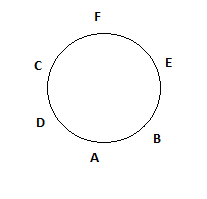Hence, A is fourth to the left of A.
Therefore, option D is the correct answer.

The data given to us is:
A is to the right of B and E is to the left of C and right of A. B is to the right of D.A is to the left of E.

This can be represented in diagrammatic form as: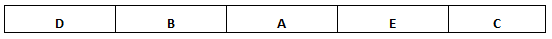Therefore, A is in the middle.
The correct option is option A.

From the figure below we can see that conclusion I is false. As the subset wireless is a subset of devices and overlaps with the set phones, the set devices must overlap with phones. Hence, some devices are phones.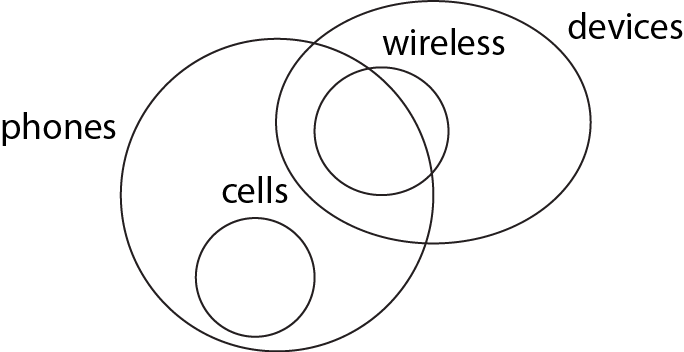As musicians is a subset of music lovers and music lovers set and ‘people who like drums’ set does not overlap => musicians and ‘people who like drums’ set cannot overlap. Hence, conclusion I follows. From the figure below we can see that conclusion II is untrue.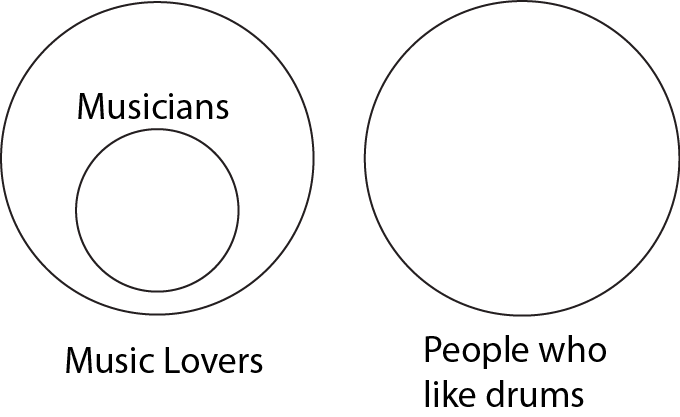The circle on the top right is entirely Rock Stars. C is only Rock Stars. F is rockstars who are also Hip Stars. B is Rockstars who are also Pop Stars. E is all the three. So, (d) is the answer..

The area of intersection of the two circles at the top is the area of interest. So, the answer is B.